The size of two internal angles of a triangle ABC are α=6/18π and β=7/18π.

Calculate the size of the third angle.

Result

γ =  0.873 rad

#### Solution:Leave us a comment of example and its solution (i.e. if it is still somewhat unclear...):Be the first to comment!## Next similar examples:

1. Angles in triangle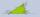Calculate the alpha angle in the triangle if beta is 61 degrees and 98 gamma degrees.
2. Chord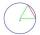Point on the circle is the end point of diameter and end point of chord length of radius. What angle between chord and diameter?
3. Two anglesThe triangles ABC and A'B'C 'are similar. In the ABC triangle, the two angles are 25° and 65°. Explain why in the triangle A'B'C 'is the sum of two angles of 90 degrees.
4. 3-bracket 3Two angles in a triangle are 90° and 60°. Has triangle at least two equal sides?
5. 3-bracketMay be the largest angle in the triangle less than 20°?
6. DiagonalCan a rhombus have the same length diagonal and side?
7. QuizQ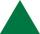An isosceles triangle has two sides of length 7 km and 39 km. How long is a third side?
8. MidpointsTriangle whose sides are midpoints of sides of triangle ABC has a perimeter 45. How long is perimeter of triangle ABC?
9. Parallelogram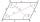In the parallelogram we know one internal angle 67°33`. Calculate the other internal angles.
10. Angles 1It is true neighboring angles have not common arm?
11. Complementary angles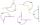Two angles are complementary. If one angle measures 10°, what is the measure of the second angle?
12. Rectangular trapezoid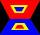How many inner right angles has a rectangular trapezoid?
13. Rhombus anglesIf one angle in the rhombus is 159°, what is it neighboring angle in rhombus?
14. Obtuse angleWhich obtuse angle is creating clocks at 17:00?
15. 8 degrees8 degrees Celsius it cools down by 9 degrees Celsius. What is the resultant temperature?
16. On SundayOn Sunday the temperature reached a high of 38° above 0. That night it dropped to 23° below 0. What is the difference between the high and low temperatures for Sunday?
17. Bitoo and ReenaBitoo ate 3/5 part of an apple and the remaining part was eaten by his sister Reena. How much part of an apple did Renna eat? Who had the larger share? By how much?